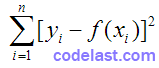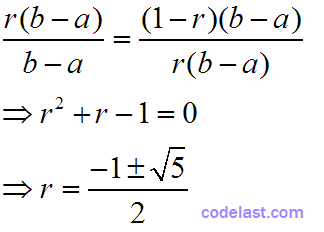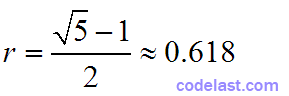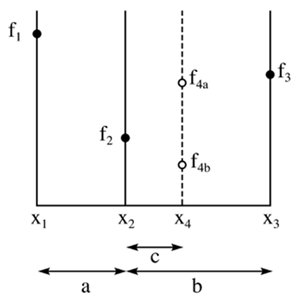（1）什么是“搜索方向”

Read More

## [原创]最小二乘的理论依据

在做数据建模或者曲线拟合的时候，我们通常会用到最小二乘法。假设作为数学模型的函数为 $y = f(x,S)$ ，其中 $S$ 为参数集向量（即一系列的参数）， $x$ 为自变量。在这种情况下，为了求出 $S$ ，需要对下式进行极小化：即：对已知的一个数据集 ${x_i}(i = 1,2, \cdots ,n)$ ，能极小化该式的 $S$ 就是最优参数。但是这个式子是怎么来的呢？Read More

## [原创]黄金比例搜索算法（Golden Section Search）的实现

<定义>数学上，集合 S 的内部（又称开核）含有所有直观上“不在 S 的边界上”的 S 的点。S 的内部中的点称为 S 的内点。

（1） $f(c) \le f(d)$ ，则极小值出现在子区间 $[a,d]$ 中，所以我们用d代替b，继续在子区间 $[a,d]$ 中搜索；

（2） $f(d) < f(c)$ ，则极小值出现在子区间 $[c,b]$ 中，所以我们用c代替a，继续在子区间 $[a,c]$ 中搜索。

（3）c=a+(1-r)(b-a)=ra+(1-r)b

（4）d=b-(1-r)(b-a)=(1-r)a+rb${x_1},{x_2},{x_3}$ 是已知的三个点（函数值“高→低→高”），它们划界了一个单峰区间，函数值分别为 ${f_1},{f_2},{f_3}$ 。现在的问题是：如何通过一个新点 ${x_4}$ ，不断缩小该区间的范围，从而逼近极小值点？

${x_4}$ 的函数值为图中 ${f_{4a}}$ 所示时（即

  public static double PHI = (1 + Math.
Read More

## [原创]LM(Levenberg-Marquard)算法的实现

LM算法，全称为Levenberg-Marquard算法，它可用于解决非线性最小二乘问题，多用于曲线拟合等场合。

LM算法的实现并不算难，它的关键是用模型函数 $f$ 对待估参数向量 $p$ 在其邻域内做线性近似，忽略掉二阶以上的导数项，从而转化为线性最小二乘问题，它具有收敛速度快等优点。LM算法属于一种“信赖域法”——所谓的信赖域法，此处稍微解释一下：在最优化算法中，都是要求一个函数的极小值，每一步迭代中，都要求目标函数值是下降的，而信赖域法，顾名思义，就是从初始点开始，先假设一个可以信赖的最大位移 $s$ ，然后在以当前点为中心，以 $s$ 为半径的区域内，通过寻找目标函数的一个近似函数（二次的）的最优点，来求解得到真正的位移。在得到了位移之后，再计算目标函数值，如果其使目标函数值的下降满足了一定条件，那么就说明这个位移是可靠的，则继续按此规则迭代计算下去；如果其不能使目标函数值的下降满足一定的条件，则应减小信赖域的范围，再重新求解。